Theory and Modern Applications

# Regarding the dynamics of a third order nonlinear difference equation

## Abstract

In this work, we study qualitative properties of the solutions of the following class of nonlinear third order difference equations

${x}_{n+1}=p{x}_{n-1}+f\left({x}_{n-1}-{x}_{n-2}\right).$

First we study the relation of attractivity and stability of equilibrium point of this equation and some related equations. Further more we prove the existence of Neimark-Sacker and period doubling (flip) bifurcation for this system by analysing the characteristic equation, and investigate the direction of this bifurcations by using normal form theory. Finally some numerical simulations are carried out to support the analytical results.

## 1 Introduction

We consider the third order difference equation

${x}_{n+1}=p{x}_{n-1}+f\left({x}_{n-1}-{x}_{n-2}\right),$
(1.1)

where $p\in \left(0,1\right)$, $f:\mathbb{R}\to \mathbb{R}$ is a continuous real function with $f\left(0\right)=0$, $f\left(x\right)\ne 0$ for $x\ne 0$ and ${x}_{0}$, ${x}_{-1}$, ${x}_{-2}$ are given real numbers (initial conditions).

Particular cases of (1.1) have been appeared in mathematical models of macroeconomics, see [1, 2]. Equations of the form ${x}_{n+1}=p{x}_{n}+f\left({x}_{n}-{x}_{n-1}\right)$ considered and studied extensively by .

In this work we study various properties of (1.1). In Section 2 we study attractivity, stability and attractive region of (1.1) and its related equations. Further more we study bifurcations of (1.1). In Section 3 we prove the existence of Neimark-Sacker and period doubling (flip) bifurcation for this system by analysing the characteristic equation, and then in Section 4 we investigate the direction of this bifurcations by using normal form theory. Finally in Section 5 we give numerical simulations to support our theoretical analysis.

## 2 Attractivity

In this section we study global attractivity and stability of the equilibrium point of (1.1). Equation (1.1) can be transformed to another form which has equivalent properties. Let

${u}_{n}={x}_{n-1}-{x}_{n-2}.$
(2.1)

Then (1.1) reduced to

${u}_{n+1}=p{u}_{n-1}+f\left({u}_{n-1}\right)-f\left({u}_{n-2}\right)$
(2.2)

which has the unique equilibrium $\overline{u}=0$.

At first we show the following result.

Theorem 2.1 The equilibrium point $\overline{x}=0$ is global attractive (respectively asymptotically stable) in (1.1) if and only if $\overline{u}=0$ is global attractive (respectively asymptotically stable) in (2.2).

Proof Equation (1.1) can be written as:

(2.3)

Hence we see that: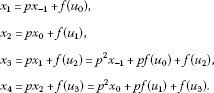Using induction we have that, if n is even then:

${x}_{n}={p}^{\frac{n}{2}}{x}_{0}+\sum _{i=1}^{\frac{n}{2}}{p}^{\frac{n}{2}-i}f\left({u}_{2i-1}\right).$
(2.4)

And if n is odd then:

${x}_{n}={p}^{\frac{n+1}{2}}{x}_{-1}+\sum _{i=1}^{\frac{n+1}{2}}{p}^{\frac{n+1}{2}-i}f\left({u}_{2i-2}\right).$
(2.5)

Now if n is even, let ${\overline{u}}_{n}={\sum }_{i=1}^{\frac{n}{2}}{p}^{\frac{n}{2}-i}|f\left({u}_{2i-1}\right)|$ and if n is odd, ${\overline{v}}_{n}={\sum }_{i=1}^{\frac{n+1}{2}}{p}^{\frac{n+1}{2}-i}|f\left({u}_{2i-2}\right)|$ we prove that ${lim}_{n⟶\mathrm{\infty }}{\overline{u}}_{n}=0$.

We distinguish two cases.

Case 1. (${\sum }_{i=1}^{\mathrm{\infty }}|f\left({u}_{2i-1}\right)|/{p}^{i}<\mathrm{\infty }$). In this case

$\underset{n⟶\mathrm{\infty }}{lim}{\overline{u}}_{n}=\underset{n⟶\mathrm{\infty }}{lim}{p}^{\frac{n}{2}}\sum _{i=1}^{\frac{n}{2}}\frac{|f\left({u}_{2i-1}\right)|}{{p}^{i}}=0.$

Case 2. (${\sum }_{i=1}^{\mathrm{\infty }}|f\left({u}_{2i-1}\right)|/{p}^{i}=\mathrm{\infty }$). In this case by using Stolz Theorem and since n is even, we have that:

$\begin{array}{rcl}\underset{n⟶\mathrm{\infty }}{lim}{\overline{u}}_{n}& =& \underset{n⟶\mathrm{\infty }}{lim}\frac{{\sum }_{i=1}^{\frac{n}{2}}\frac{|f\left({u}_{2i-1}\right)|}{{p}^{i}}}{\frac{1}{{p}^{\frac{n}{2}}}}\\ =& \underset{n⟶\mathrm{\infty }}{lim}\frac{{\sum }_{i=1}^{\frac{n+2}{2}}\frac{|f\left({u}_{2i-1}\right)|}{{p}^{i}}-{\sum }_{i=1}^{\frac{n}{2}}\frac{|f\left({u}_{2i-1}\right)|}{{p}^{i}}}{\frac{1}{{p}^{\frac{n+2}{2}}}-\frac{1}{{p}^{\frac{n}{2}}}}\\ =& \underset{n⟶\mathrm{\infty }}{lim}\frac{|f\left({u}_{n+1}\right)|}{1-p}.\end{array}$

Using continuity of f we see that if ${lim}_{n⟶\mathrm{\infty }}{u}_{n}=0$ then ${lim}_{n⟶\mathrm{\infty }}{\overline{u}}_{n}=0$ (and by a similar argument ${lim}_{n⟶\mathrm{\infty }}{\overline{v}}_{n}=0$), which implies that ${lim}_{n⟶\mathrm{\infty }}{x}_{n}=0$. □

By using weak contractions introduced in  we obtain the following sufficient conditions for attractivity of solutions of (1.1).

Proposition 2.2

1. (1)

If $|f\left(t\right)|\le a|t|$ for all t and $0, then origin is globally attracting in (1.1).

2. (2)

If $0 for all t and $0, then every positive solution of (1.1) converges to zero.

Proof For the proof of (1), define $F\left({y}_{1},{y}_{2},{y}_{3}\right)=p{y}_{2}+f\left({y}_{2}-{y}_{1}\right)$ and notice that

$|F\left({y}_{1},{y}_{2},{y}_{3}\right)|\le p|{y}_{2}|+a|{y}_{2}-{y}_{1}|\le \left(p+2a\right)max\left\{|{y}_{1}|,|{y}_{2}|,|{y}_{3}|\right\}$

since $p+2a<1$, it follows that F is a weak contraction on the entire space and therefore by , the origin is globally attracting.

1. (2)

For ${y}_{1},{y}_{2},{y}_{3}\ge 0$ notice that:

$F\left({y}_{1},{y}_{2},{y}_{3}\right)\le p{y}_{2}+amax\left\{{y}_{1},{y}_{2}\right\}\le \left(p+a\right)max\left\{{y}_{1},{y}_{2},{y}_{3}\right\}.$

Now since $p+a<1$, it follows that F is a weak contraction on $\left[0,1{\right)}^{3}$, and since $\left[0,1{\right)}^{3}$ is invariant under ${V}_{F}\left({y}_{1},{y}_{2},{y}_{3}\right)=\left(g\left({y}_{1},{y}_{2},{y}_{3}\right),{y}_{1},{y}_{2}\right)$,  implies that the origin is exponentially stable relative to $\left[0,1{\right)}^{3}$, hence every positive solution of (1.1) converges to zero. □

Now we study stability properties. Let ${x}_{n}$ be a solution of (1.1). We define the vector $y\left(n\right)=\left({y}_{1}\left(n\right),{y}_{2}\left(n\right),{y}_{3}\left(n\right)\right)\in {\mathbb{R}}^{3}$, where

${y}_{j}\left(n\right)={x}_{n+j-3},\phantom{\rule{1em}{0ex}}j=1,2,3.$
(2.6)

Using this notation the delay Equation (1.1) transformed to the following 3D system:

$y\left(n+1\right)=g\left(y\left(n\right)\right),$
(2.7)

where $g\left(y\right)=\left({y}_{2},{y}_{3},p{y}_{2}+f\left({y}_{2}-{y}_{1}\right)\right)$ which has the unique equilibrium point $\left(0,0,0\right)$.

Now we study the relation of the stability properties of the delay Equation (1.1) to those of the associated nondelay equation:

${x}_{n+1}=f\left({x}_{n}\right),\phantom{\rule{1em}{0ex}}n\ge -1.$
(2.8)

First we prove the following lemma which will be used in the next results.

Lemma 2.3 Let $y\left(n\right)$ be a solution of the system (2.7). Then for $n\ge 4-j$, the following statements are true:

For odd $n+j$:

$|{y}_{j}\left(n\right)|\le {p}^{\frac{n+j-2}{2}}|{y}_{2}\left(0\right)|+\sum _{i=1}^{\frac{n+j-2}{2}}{p}^{\frac{n+j-2}{2}-i}|f\left({y}_{2}\left(2i-2\right)-{y}_{1}\left(2i-2\right)\right)|.$
(2.9)

And for even $n+j$:

$|{y}_{j}\left(n\right)|\le {p}^{\frac{n+j-3}{2}}|{y}_{3}\left(0\right)|+\sum _{i=1}^{\frac{n+j-3}{2}}{p}^{\frac{n+j-3}{2}-i}|f\left({y}_{2}\left(2i-1\right)-{y}_{1}\left(2i-1\right)\right)|.$
(2.10)

Furthermore for $0\le n\le 3-j$:

${y}_{j}\left(n\right)={y}_{j+n}\left(0\right).$
(2.11)

Proof From (2.6) we have that for $j=1,2,3$: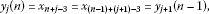(2.12)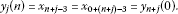(2.13)

Now by using these relations and induction we see that, if n is odd then:

${y}_{3}\left(n\right)={p}^{\frac{n+1}{2}}{y}_{2}\left(0\right)+\sum _{i=1}^{\frac{n+1}{2}}{p}^{\frac{n+1}{2}-i}f\left({y}_{2}\left(2i-2\right)-{y}_{1}\left(2i-2\right)\right).$
(2.14)

And if n is even then:

${y}_{3}\left(n\right)={p}^{\frac{n}{2}}{y}_{3}\left(0\right)+\sum _{i=1}^{\frac{n}{2}}{p}^{\frac{n}{2}-i}f\left({y}_{2}\left(2i-1\right)-{y}_{1}\left(2i-1\right)\right).$
(2.15)

Furthers more:

${y}_{j}\left(n\right)={x}_{n+j-3}={x}_{\left(n+j-3\right)+3-3}={y}_{3}\left(n+j-3\right).$
(2.16)

By using this relation we have that, if $n+j$ is odd then:

${y}_{j}\left(n\right)={y}_{3}\left(n+j-3\right)={p}^{\frac{n+j-2}{2}}{y}_{2}\left(0\right)+\sum _{i=1}^{\frac{n+j-2}{2}}{p}^{\frac{n+j-2}{2}-i}f\left({y}_{2}\left(2i-2\right)-{y}_{1}\left(2i-2\right)\right).$

And if $n+j$ is even then:

${y}_{j}\left(n\right)={y}_{3}\left(n+j-3\right)={p}^{\frac{n+j-3}{2}}{y}_{3}\left(0\right)+\sum _{i=1}^{\frac{n+j-3}{2}}{p}^{\frac{n+j-3}{2}-i}f\left({y}_{2}\left(2i-1\right)-{y}_{1}\left(2i-1\right)\right).$

□

Theorem 2.4 Assume that f satisfies:

$|f\left(x+y\right)|\le |f\left(x\right)|+|f\left(y\right)|$
(2.17)

for all $x,y\in \mathbb{R}$. If the equilibrium point of (2.8) is stable, then the equilibrium point of (1.1) is also stable.

Proof It is sufficient to prove the stability of the equilibrium of (2.7) because of the equivalence of (1.1) and (2.7). Let $\epsilon >0$ be arbitrary. Since the equilibrium point of (2.8) is stable, there exists ${\delta }_{1}>0$ such that $|{x}_{-2}|<{\delta }_{1}$ implies $|{x}_{n}|<\frac{\left(1-p\right)\epsilon }{2}$ for all $n\ge -2$. Choose $\delta =min\left({\delta }_{1},\frac{\left(1-p\right)\epsilon }{2}\right)$, since $y\left(0\right)=\left({y}_{1}\left(0\right),{y}_{2}\left(0\right),{y}_{3}\left(0\right)\right)=\left({x}_{-2},{x}_{-1},{x}_{0}\right)$, we have that

$\parallel y\left(0\right)\parallel =max\left(|{y}_{1}\left(0\right)|,|{y}_{2}\left(0\right)|,|{y}_{3}\left(0\right)|\right)=max\left(|{x}_{-2}|,|{x}_{-1}|,|{x}_{0}|\right)\le \delta \le {\delta }_{1}.$

Now for $n\ge -2$:

$|{x}_{n}|\le \frac{\left(1-p\right)\epsilon }{2}$
(2.18)

which implies that:

$|f\left({x}_{n}\right)|\le \frac{\left(1-p\right)\epsilon }{2}$
(2.19)

for all $n\ge -1$. Therefore, for $n\ge 0$:

$|f\left({y}_{2}\left(n\right)\right)|\le \frac{\left(1-p\right)\epsilon }{2},\phantom{\rule{2em}{0ex}}|f\left({y}_{1}\left(n\right)\right)|\le \frac{\left(1-p\right)\epsilon }{2}.$
(2.20)

And hence

$|f\left({y}_{2}\left(n\right)-{y}_{1}\left(n\right)\right)|<|f\left({y}_{2}\left(n\right)\right)|+|f\left({y}_{1}\left(n\right)\right)|<\frac{\left(1-p\right)\epsilon }{2}+\frac{\left(1-p\right)\epsilon }{2}=\left(1-p\right)\epsilon .$

Now $\parallel y\left(0\right)\parallel \le \delta$ implies that $|{y}_{j}\left(0\right)|<\delta <\frac{\left(1-p\right)\epsilon }{2}<\epsilon$ for $j=1,2,3$. Hence

and from the previous lemma, if $n+j$ is odd then:

$\begin{array}{rcl}|{y}_{j}\left(n\right)|& \le & {p}^{\frac{n+j-2}{2}}|{y}_{2}\left(0\right)|+\sum _{i=1}^{\frac{n+j-2}{2}}{p}^{\frac{n+j-2}{2}-i}|f\left({y}_{2}\left(2i-2\right)-{y}_{1}\left(2i-2\right)\right)|\\ \le & \epsilon {p}^{\frac{n+j-2}{2}}+\left(1-p\right)\epsilon {p}^{\frac{n+j-2}{2}}\sum _{i=1}^{\frac{n+j-2}{2}}{p}^{-i}=\epsilon \end{array}$

and if $n+j$ is even then:

$\begin{array}{rcl}|{y}_{j}\left(n\right)|& \le & {p}^{\frac{n+j-3}{2}}|{y}_{3}\left(0\right)|+\sum _{i=1}^{\frac{n+j-3}{2}}{p}^{\frac{n+j-3}{2}-i}|f\left({y}_{2}\left(2i-1\right)-{y}_{1}\left(2i-1\right)\right)|\\ \le & \epsilon {p}^{\frac{n+j-3}{2}}+\left(1-p\right)\epsilon {p}^{\frac{n+j-3}{2}}\sum _{i=1}^{\frac{n+j-3}{2}}{p}^{-i}=\epsilon .\end{array}$

Therefore, for arbitrary $\epsilon >0$, there exists $\delta >0$, such that $\parallel y\left(0\right)\parallel <\delta$ implies that $\parallel y\left(n\right)\parallel <\epsilon$ for $n\ge 0$, and hence the equilibrium point of (2.7) is stable. □

Theorem 2.5 Assume that (2.17) holds. If there exists $m>0$ such that $G\left(m\right)=\left\{x\in \mathbb{R}:|x| is a subset of the attractive region of the equilibrium point of (2.8), then $G\left(m\right)$ is also contained in the attractive region of the equilibrium point of (1.1).

Proof Let $\epsilon >0$ be arbitrary. Since $G\left(m\right)$ is a subset of attractive region of (2.8), there exists ${T}_{1}$ such that $|{x}_{-1}| implies that $|{x}_{n}|<\epsilon$ for $n>{T}_{1}$. Assume that $\parallel y\left(0\right)\parallel , then we have $|{x}_{-1}|. So there exists ${T}_{2}\ge {T}_{1}$ such that $|x\left(n\right)|<\left(1-p\right)\epsilon /4$ for $n\ge {T}_{2}$, which implies that:

$\begin{array}{rcl}|f\left({y}_{2}\left(n\right)-{y}_{1}\left(n\right)\right)|& \le & |f\left({y}_{2}\left(n\right)\right)|+|f\left({y}_{1}\left(n\right)\right)|\\ <& \frac{\left(1-p\right)\epsilon }{4}+\frac{\left(1-p\right)\epsilon }{4}\le \frac{\left(1-p\right)\epsilon }{2}\end{array}$

for all $n\ge {T}_{2}+1$. Let $j=1,2,3$. If $n+j$ is odd we have:

$\begin{array}{rcl}|{y}_{j}\left(n\right)|& \le & {p}^{\frac{n+j-2}{2}}|{y}_{2}\left(0\right)|\\ +\sum _{i=1}^{\frac{n+j-2}{2}}{p}^{\frac{n+j-2}{2}-i}|f\left({y}_{2}\left(2i-2\right)-{y}_{1}\left(2i-2\right)\right)|\\ <& m{p}^{\frac{n+j-2}{2}}+\sum _{i=1}^{{T}_{2}+1}{p}^{\frac{n+j-2}{2}-i}|f\left({y}_{2}\left(2i-2\right)-{y}_{1}\left(2i-2\right)\right)|+\frac{\epsilon }{2}\end{array}$

provided that $n\ge 4-j$. If $n+j$ is even we have:

$\begin{array}{rcl}|{y}_{j}\left(n\right)|& \le & {p}^{\frac{n+j-3}{2}}|{y}_{3}\left(0\right)|\\ +\sum _{i=1}^{\frac{n+j-3}{2}}{p}^{\frac{n+j-3}{2}-i}|f\left({y}_{2}\left(2i-1\right)-{y}_{1}\left(2i-1\right)\right)|\\ <& m{p}^{\frac{n+j-3}{2}}+\sum _{i=1}^{{T}_{2}+1}{p}^{\frac{n+j-3}{2}-i}|f\left({y}_{2}\left(2i-1\right)-{y}_{1}\left(2i-1\right)\right)|+\frac{\epsilon }{2}.\end{array}$

Now:

$|f\left({y}_{2}\left(i\right)-{y}_{1}\left(i\right)\right)|\le |f\left({x}_{i-2}\right)|+|f\left({x}_{i-1}\right)|\le |{f}^{i}\left({x}_{-1}\right)|+|{f}^{i+1}\left({x}_{-1}\right)|.$
(2.21)

The continuity of f implies there exists $L>0$ such that $|{f}^{i}\left({x}_{-1}\right)| and $|{f}^{i+1}\left({x}_{-1}\right)|. Now if $n+j$ is odd we have for $n\ge {T}_{2}+2$:

$\begin{array}{rcl}|{y}_{j}\left(n\right)|& <& m{p}^{\frac{n+j-2}{2}}+\sum _{i=1}^{{T}_{2}+1}{p}^{\frac{n+j-2}{2}-i}|f\left({y}_{2}\left(2i-2\right)-{y}_{1}\left(2i-2\right)\right)|+\frac{\epsilon }{2}\\ <& \frac{\epsilon }{2}+\left(m+\frac{2L}{1-p}\right){p}^{\frac{n+j-2{T}_{2}-4}{2}}.\end{array}$

Now choose ${T}_{3}$ such that:

$\left(m+\frac{2L}{1-p}\right){p}^{\frac{n+j-2{T}_{2}-4}{2}}\le \frac{\epsilon }{2}$
(2.22)

holds for $n\ge {T}_{3}$.

If $n+j$ is even we have:

$\begin{array}{rcl}|{y}_{j}\left(n\right)|& <& m{p}^{\frac{n+j-3}{2}}+\sum _{i=1}^{{T}_{2}+1}{p}^{\frac{n+j-3}{2}-i}|f\left({y}_{2}\left(2i-1\right)-{y}_{1}\left(2i-1\right)\right)|+\frac{\epsilon }{2}\\ <& \frac{\epsilon }{2}+\left(m+\frac{2L}{1-p}\right){p}^{\frac{n+j-2{T}_{2}-5}{2}}.\end{array}$

Now choose ${T}_{4}$ such that:

$\left(m+\frac{2L}{1-p}\right){p}^{\frac{n+j-2{T}_{2}-5}{2}}\le \frac{\epsilon }{2}$
(2.23)

holds for $n\ge {T}_{4}$. We consider ${T}_{5}=min\left({T}_{3},{T}_{4}\right)$, Then $\parallel y\left(0\right)\parallel implies that $\parallel y\left(n\right)\parallel <\epsilon$ for $n\ge {T}_{5}$. Hence $G\left(m\right)$ is also subset of attractive region of the equilibrium of (1.1). □

## 3 Existence of bifurcations

Now we study bifurcations of (1.1), for this aim we suppose that $f\in {C}^{3}$. First we prove the existence of bifurcations. Dynamics of system (1.1) described by the mapping:

$g\left({y}_{1},{y}_{2},{y}_{3}\right)=\left({y}_{2},{y}_{3},p{y}_{2}+f\left({y}_{2}-{y}_{1}\right)\right).$
(3.1)

The Jacobian matrix of g at O is:

$H=D{g}_{{|}_{O}}=\left[\begin{array}{ccc}0& 1& 0\\ 0& 0& 1\\ -q& p+q& 0\end{array}\right]$

in which $q={f}^{\prime }\left(0\right)$. The characteristic equation of H is:

${\lambda }^{3}-\left(p+q\right)\lambda +q=0.$
(3.2)

By the Jury’s conditions, the necessary and sufficient conditions for all eigenvalues of the characteristic equation lying inside the unit circle are:

$\left\{\begin{array}{c}p+2q-1<0,\hfill \\ p-1<0,\hfill \\ 3+p-2q>0,\hfill \\ 4q+p+3>0,\hfill \\ p+q-{q}^{2}+1>0.\hfill \end{array}$

We consider the following curves, which are the boundary curves of the region of asymptotic stability shown in Figure 1:

$\left\{\begin{array}{cc}{L}_{1}:p+2q-1=0,\hfill & 0

On ${L}_{2}$, ${L}_{4}$, respectively $p=1,0$ which is impossible. We show in the following theorem occurrence of bifurcations on the boundary curves ${L}_{1}$, ${L}_{3}$.

Theorem 3.1 For system (3.1) the following conditions holds:

1. (1)

Flip bifurcation occurs when $\left(p,q\right)\in {L}_{1}$.

2. (2)

Neimark-Sacker bifurcation occurs when $\left(p,q\right)\in {L}_{3}$.

Proof First, we show the existence of the flip bifurcation. Because $\left(p,q\right)\in {L}_{1}$, we have the characteristic equation:

$\left(\lambda +1\right)\left({\lambda }^{2}-\lambda +q\right)=0$
(3.3)

which has eigenvalues ${\lambda }_{1}=-1$, ${\lambda }_{2,3}=\left(\frac{1±\sqrt{1-4q}}{2}\right)$. So $Y=\left(1,-1,1\right)$ is an eigenvector of H with corresponding eigenvalue ${\lambda }_{1}=-1$, and 1 is not the eigenvalue. A straight forward calculation shows that:

$\begin{array}{c}Range{\left(I+Dg\left({X}^{\ast }\right)\right)}_{{|}_{2q+p=1}}\hfill \\ \phantom{\rule{1em}{0ex}}=Span{\left(x+y,y+z,\left(p+q-1\right)x+\left(p+q\right)y+z\right)}^{T}\hfill \end{array}$

in which $\left(x,y,z\right)\in {\mathbb{R}}^{3}$. Now:

$\frac{d}{dq}Dg{\left({X}^{\ast }\right)}_{{|}_{2q+p=1}}=\left[\begin{array}{ccc}0& 0& 0\\ 0& 0& 0\\ -1& 1& 0\end{array}\right]$

and:

$\frac{d}{dq}Dg{\left({X}^{\ast }\right)}_{{|}_{2q+p=1}}Y=\left(0,0,-2\right)\notin Range{\left(I+Dg\left({X}^{\ast }\right)\right)}_{{|}_{2q+p=1}}.$

Therefore by , Th.1.4.5], flip bifurcation occurs.

Now we show the existence of Neimark-Sacker bifurcation. If $\lambda ={e}^{i\theta }$ is a root of Equation (3.2), separating the real and imaginary parts, we have the following:

$\left\{\begin{array}{c}cos3\theta -\left(p+q\right)cos\theta =-q,\hfill \\ sin3\theta -\left(p+q\right)sin\theta =0\hfill \end{array}$

squaring and adding both equations, we have:

$1-{\left(p+q\right)}^{2}-2\left(p+q\right)cos2\theta ={q}^{2}$

so we get:

$cos2\theta =\frac{1+{\left(p+q\right)}^{2}-{q}^{2}}{2\left(p+q\right)}.$

In the case that $\left(p,q\right)\in {L}_{3}$ we have $q+p={q}^{2}-1$. By substitution of $q+p={q}^{2}-1$ into $\frac{1+{\left(p+q\right)}^{2}-{q}^{2}}{2\left(p+q\right)}$, we have:

$cos2\theta =\frac{{q}^{2}-2}{2}.$

Therefore, Equation (3.2) has a unique pair of complex roots:

${\lambda }_{1,2}={e}^{±i\left(\frac{arc cos\frac{{q}^{2}-2}{2}}{2}\right)}.$

On the other hand, $-1 means $-1. Because $cos2\theta =2{cos}^{2}\theta -1$ and $-1, we get $-1<2{cos}^{2}\theta -1<\frac{-1}{2}$ and hence $0<|cos\theta |<\frac{1}{2}$, which refers $arg\lambda \ne 0,±\frac{\pi }{2},±\frac{2\pi }{3},±\pi$. Thus ${\lambda }^{k}\ne 1$, for . On the other hand we have:

$\begin{array}{rcl}{\left(\frac{d{|\lambda |}^{2}}{dq}\right)}_{|p+q-{q}^{2}+1=0}& =& {\left(\overline{\lambda }\frac{d\lambda }{dq}+\lambda \frac{d\overline{\lambda }}{dq}\right)}_{|p+q-{q}^{2}+1=0}\\ =& \frac{2\left(1-cos\theta \right)\left(12{cos}^{2}\theta +6cos\theta -\left(p+q\right)-3\right)}{{|3{e}^{2i\theta }-\left(p+q\right)|}^{2}}.\end{array}$

Assume that ${\left(\frac{d{|\lambda |}^{2}}{dq}\right)}_{|p+q-{q}^{2}+1=0}=0$, that is, $cos\theta =1$ or $12{cos}^{2}\theta +6cos\theta -\left(p+q\right)-3=0$. In previous discussion, we obtain $0, hence $cos\theta \ne 1$. If $12{cos}^{2}\theta +6cos\theta -\left(p+q\right)-3=0$, then from $cos2\theta =\frac{{q}^{2}-2}{2}$ and $p+q={q}^{2}-1$, we see that $q=±2,±\frac{1}{2}$, which corresponds to $p=1,5,-\frac{5}{4},-\frac{1}{4}$ that is impossible on ${L}_{3}$. So we have that, ${\left(\frac{d{|\lambda |}^{2}}{dq}\right)}_{|p+q-{q}^{2}+1=0}\ne 0$. Therefore, by the generic Neimark-Sacker bifurcation theorem [7, 8], Neimark-Sacker bifurcation occurs, that is, the system (3.1) has a unique closed invariant curve bifurcating from the equilibrium ${X}^{\ast }$. □

## 4 Direction of the bifurcations

In the previous section, we have shown that system (3.1) undergoes a flip (period-doubling) bifurcation when $\left(p,q\right)\in {L}_{1}$ and a Neimark-Sacker bifurcation when $\left(p,q\right)\in {L}_{3}$ at equilibrium point ${X}^{\ast }$. In this section, by using the normal form method for discrete systems, as studied by Sacker, Kuznetsov and Wiggins, we shall study the direction of the two bifurcations and stability of the bifurcating invariant curves. We can write system as:

${U}_{n+1}=D{U}_{n}+G\left({U}_{n}\right),\phantom{\rule{1em}{0ex}}{U}_{n}\in {\mathbb{R}}^{3},$
(4.1)

where $G\left(U\right)=O\left({\parallel U\parallel }^{3}\right)$ is a smooth function and its Taylor expansion is:

$G\left(U\right)=\frac{1}{2}B\left(U,U\right)+\frac{1}{6}C\left(U,U,U\right)+O\left({\parallel U\parallel }^{3}\right),$
(4.2)

where:

$B\left(U,U\right)=\left(0,0,{b}_{3}\left(U,U\right)\right),\phantom{\rule{2em}{0ex}}C\left(U,U,U\right)=\left(0,0,{c}_{3}\left(U,U,U\right)\right)$

in which:

$\left\{\begin{array}{c}{b}_{3}\left(\varphi ,\psi \right)={f}^{″}\left(0\right)\left({\varphi }_{1}{\psi }_{1}-{\varphi }_{2}{\psi }_{2}\right),\hfill \\ {c}_{1}\left(\varphi ,\psi ,\eta \right)={c}_{3}\left(\varphi ,\psi ,\eta \right)={f}^{‴}\left(0\right)\left(-{\varphi }_{1}{\psi }_{1}{\eta }_{1}+{\varphi }_{1}{\psi }_{2}{\eta }_{1}-{\varphi }_{2}{\psi }_{2}{\eta }_{1}\right)\hfill \end{array}$

for $\varphi =\left({\varphi }_{1},{\varphi }_{2},{\varphi }_{3}\right)\in {\mathbb{R}}^{3}$, $\psi =\left({\psi }_{1},{\psi }_{2},{\psi }_{3}\right)\in {\mathbb{R}}^{3}$ and $\eta =\left({\eta }_{1},{\eta }_{2},{\eta }_{3}\right)\in {\mathbb{R}}^{3}$. At the beginning, we study the direction of period-doubling bifurcation and the stability of period-doubling cycle. Let $w\in {\mathbb{R}}^{3}$ be the eigenvector of H with respect to eigenvalue −1, that is, $Hw=-w$; $v\in {\mathbb{R}}^{3}$ be the adjoint eigenvector of ${H}^{T}$ that is, ${H}^{T}v=-v$ where ${H}^{T}$ is the transposed matrix, and $〈v,w〉=1$, where $〈\cdot ,\cdot 〉$ is the standard scalar product in ${\mathbb{R}}^{3}$. So we have:

$\left\{\begin{array}{c}w=\left(-1,1,-1\right),\hfill \\ v=\frac{-1}{q+2}\left(q,-1,1\right).\hfill \end{array}$

Following the algorithms given in Kuznetsov , the critical normal form coefficient $c\left(0\right)$, that determines the nondegeneracy of the period-doubling bifurcation and the stability of period-doubling cycle, is given by the following formula:

$c\left(0\right)=\frac{1}{6}〈v,C\left(w,w,w\right)〉-\frac{1}{2}〈v,B\left(w,{\left(H-I\right)}^{-1}B\left(w,w\right)\right)〉.$
(4.3)

From the above relations we have:

$\left\{\begin{array}{c}〈v,B\left(w,{\left(H-I\right)}^{-1}B\left(w,w\right)\right)〉=0,\hfill \\ 〈v,C\left(w,w,w\right)〉=\frac{16{f}^{‴}\left(0\right)}{1-p}\hfill \end{array}$

and therefore:

$c\left(0\right)=\frac{8{f}^{‴}\left(0\right)}{3\left(1-p\right)}.$

Applying the general theory for the direction of flip bifurcation and stability of period doubling cycle (see Wiggins  or Kuznetsov ), we derive the following result:

Theorem 4.1 For system (3.1) flip bifurcation occurs in ${X}^{\ast }$ when $p+2q=1$, and if ${f}^{‴}\left(0\right)>0$, the flip bifurcation is supercritical and if ${f}^{‴}\left(0\right)<0$, the flip bifurcation is subcritical.

Now, we are going to study the direction of the Neimark-Sacker bifurcation and the stability of the bifurcating invariant curve in ${X}^{\ast }$. In the above section, we see that H has simple eigenvalues on the unit circle:

${\lambda }_{1,2}={e}^{±i{\theta }_{0}},\phantom{\rule{2em}{0ex}}{\theta }_{0}=\frac{arc cos\frac{1+{\left(p+q\right)}^{2}-{q}^{2}}{2\left(p+q\right)}}{2}.$

Let α be a complex eigenvector corresponding to ${e}^{i\theta }$ and β be a complex eigenvector of the transposed matrix ${H}^{T}$ corresponding ${e}^{-i\theta }$, i.e. $H\alpha ={e}^{i{\theta }_{0}}\alpha$, ${H}^{T}\beta ={e}^{-i{\theta }_{0}}\beta$. By computation we obtain the following eigenvectors:

$\alpha ={\left(1,{e}^{i{\theta }_{0}},{e}^{2i{\theta }_{0}}\right)}^{T},\phantom{\rule{2em}{0ex}}\beta ={\left(1,\frac{-1}{q}{e}^{-2i{\theta }_{0}},\frac{-1}{q}{e}^{-i{\theta }_{0}}\right)}^{T}.$

Normalize α with respect to β such that:

$〈\beta ,\alpha 〉=1,$

where $〈\cdot ,\cdot 〉$ means the standard scalar product in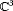defined by $〈\beta ,\alpha 〉=\overline{{\beta }_{1}}{\alpha }_{1}+\overline{{\beta }_{2}}{\alpha }_{2}+\overline{{\beta }_{3}}{\alpha }_{3}$, we have: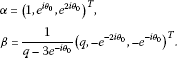Following the algorithms given in Kuznetsov , the critical normal form coefficient $a\left(0\right)$, that determines the nondegeneracy of the Neimark-Sacker bifurcation and allows us to predict the stability of bifurcating invariant curve, is given by the following formula:

$\begin{array}{rcl}a\left(0\right)& =& \frac{1}{2}Re\left({e}^{-i{\theta }_{0}}\left[〈\beta ,C\left(\alpha ,\alpha ,\overline{\alpha }\right)〉+2〈\beta ,B\left(\alpha ,{\left(I-H\right)}^{-1}B\left(\alpha ,\overline{\alpha }\right)\right)〉\\ +〈\beta ,B\left(\overline{\alpha },{\left({e}^{2i{\theta }_{0}}I-H\right)}^{-1}\right)B\left(\alpha ,\alpha \right)〉\right]\right).\end{array}$

Furthermore in this case we have: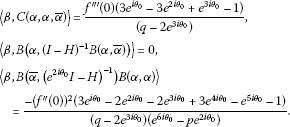Which yields the following formula for $a\left(0\right)$:

$a\left(0\right)=\frac{{f}^{‴}\left(0\right){A}_{1}+{\left({f}^{″}\left(0\right)\right)}^{2}{A}_{2}}{2\left({A}_{0}^{2}+{B}_{0}^{2}\right)}$

in which: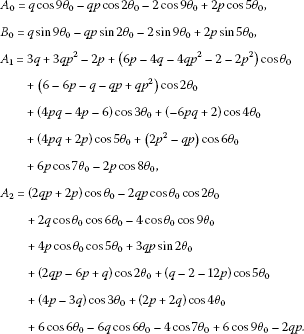From the theory of the direction of Neimark-Sacker bifurcation and the stability of the bifurcating invariant curve (see Sacker [10, 11], Wiggins  or Kuznetsov ), we have:

Theorem 4.2 For system (3.1), if $p+q-{q}^{2}+1=0$ hold, then $a\left(0\right)<0$ (respectively >0) implies that a unique and stable (respectively, unstable) closed invariant curve bifurcates from ${X}^{\ast }$, and the Neimark-Sacker bifurcation in ${X}^{\ast }$ is supercritical (respectively, subcritical).

## 5 Numerical simulations

In this section, we give numerical simulations to illustrate our theoretical analysis.

Example 1 Let $p=\frac{1}{2}$ and $f\left(t\right)=\frac{1}{4}t+{t}^{3}$. In this case $q=\frac{1}{4}$, $c\left(0\right)=32$ and $\left(p,q\right)\in {L}_{1}$, therefore by Theorem 4.1 flip bifurcation occurs. Figures 2, 3 and 4 show bifurcation diagram.

Example 2 Let $p=\frac{11}{25}$ and $f\left(t\right)=-\frac{4}{5}t+{t}^{3}$. In this case $q=-\frac{4}{5}$, $a\left(0\right)=-0.9883$, and $\left(p,q\right)\in {L}_{2}$, therefore by Theorem 4.2 Neimark-Sacker bifurcation occurs. Figures 5, 6 and 7 show orbits of system when $p=\frac{9}{25}$, $p=\frac{11}{25}$ and $p=\frac{11.9}{25}$, respectively.

## References

1. Eckaus RS: The stability of dynamic models. Rev. Econ. Stat. 1957, 39: 172–182. 10.2307/1928534

2. Hicks JR: A Contribution to the Theory of the Trade Cycle. Clarendon, Oxford; 1965.

3. Dai B, Zhang N: Stability and global attractivity for a class of nonlinear delay difference equations. Discrete Dyn. Nat. Soc. 2005, 3: 227–234.

4. Li S, Zhang W: Bifurcations in a second-order difference equation from macroeconomics. J. Differ. Equ. Appl. 2007, 2: 365–374.

5. Sedaghat H: A class of nonlinear second-order difference equations from macroeconomics. Nonlinear Anal. 1997, 29: 593–603. 10.1016/S0362-546X(96)00054-5

6. Sedaghat H: Nonlinear Difference Equations: Theory with Applications to Social Science Models. Kluwer Academic, Dordrecht; 2003.

7. Stuart AM, Humphries AR: Dynamical Systems and Numerical Analysis. Cambridge University Press, Cambridge; 1998.

8. Kuznetsov YA: Elements of Applied Bifurcation Theory. Springer, New York; 1998.

9. Wiggins S: Introduction to Applied Nonlinear Dynamical Systems and Chaos. Springer, New York; 1990.

10. Sacker, RJ: On invariant surfaces and bifurcation of periodic solutions of ordinary differential equations. Technical report IMM-NYU 333, New York University (1964)

11. Sacker RJ, Von Bremen HF: Bifurcation of maps and cycling in genetic systems. Fields Inst. Commun. 2004, 42: 305–311.

## Acknowledgements

The authors would like to thank the referees for their useful comments.

## Author information

Authors

### Corresponding author

Correspondence to Reza Memarbashi.

### Competing interests

The authors declare that they have no competing interests.

### Authors’ contributions

All authors carried out the proof. All authors conceived of the study, and participated in its design and coordination. All authors read and approved the final manuscript.

## Authors’ original submitted files for images

Below are the links to the authors’ original submitted files for images.

## Rights and permissions

Reprints and Permissions

Memarbashi, R., Ghasemabadi, A. Regarding the dynamics of a third order nonlinear difference equation. Adv Differ Equ 2012, 107 (2012). https://doi.org/10.1186/1687-1847-2012-107

• Accepted:

• Published:

• DOI: https://doi.org/10.1186/1687-1847-2012-107

### Keywords

• difference equations
• attractivity
• stability
• flip bifurcation
• Neimark-Sacker bifurcation
• macroeconomic models